# 1 Pheasant Documentation

## 1.1 Overview

Welcome to Pheasant. Pheasant is a Markdown converter which is designed to work with MkDocs as a plugin.

Highlights include:

• Auto generation of outputs for a fenced code block or inline code in Markdown source using Jupyter client. The code language is not restricted to Python.
• Auto numbering of headers, figures, tables, and etc. Numbered objects can be linked from other Markdown sources.

## 1.2 Installation

You can install Pheasant from PyPI.

``\$ pip install pheasant``

If you use Pheasant as a plugin of MkDocs, you also need to install it (version 1.1.1 or later is required).

``\$ pip install mkdocs``

In your `mkdocs.yml`, add lines below to register Pheasant as a MkDocs plugin.

``````plugins:
- pheasant``````

## 1.3 Getting Started

### 1.3.1 Auto generation of the executed outputs with Jupyter client

In a markdown fenced code below,

`````````python
print(1)
`````````

a `print` function is executed via Jupyter client and converted into HTML:

``````<div class="input"><pre><code class="python">print(1)</code></pre></div>
<div class="stdout"><pre><code class="nohighlight">1</code></pre></div>``````

Then, finally rendered as:

``print(1)``

 2020-12-20 08:15:12 (6.70ms) python3 (22.4ms)

``1``

Other language code can be executed if a kernel for the language has been installed. For example,

`````````julia
println("Hello, IJulia!")
`````````
``println("Hello, IJulia!")``

 () ()

You can check the kernel name and its total execution time during the conversion process at the right side of an input cell.

Like Jupyter Notebook, the last object in a code cell is displayed as the output of the cell.

`````````python
greeting = 'Hello, Python'
greeting
`````````
``````greeting = 'Hello, Python'
greeting``````

 2020-12-20 08:15:12 (6.25ms) python3 (28.6ms)

``'Hello, Python'``

### 1.3.2 Inline code embeded in a Markdown source

"Inline code" is a powerful feature of Pheasant. Any executable codes surrounded by `{{` and `}}` are automatically executed and the codes are replaced with the execution result. For example, `{{3*4}}` becomes 12. Variables can be assigned in an inline code like this: `{{name='Pheasant'}}`. Then, `"I'm {{name}}."` becomes "I'm Pheasant."

### 1.3.3 Visualization

Pheasant supports various output formats other than the standard stream (`stdout`/`stderr`) or a plain text output. For example, you can create a PNG image using Matplotlib. First, import the Matplotlib plotting library.

`````````python
import matplotlib.pyplot as plt
`````````
``import matplotlib.pyplot as plt``

 2020-12-20 08:15:12 (144ms) python3 (213ms)

Plot a line.

`````````python
plt.plot([1, 2])
`````````
``plt.plot([1, 2])``

 2020-12-20 08:15:12 (102ms) python3 (315ms)

``[<matplotlib.lines.Line2D at 0x211993331f0>]``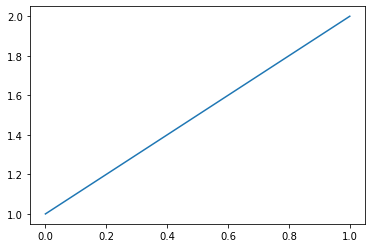Execution of the above Markdown source on a Jupyter client creates a plain text output as an execute result and a PNG image as display data. You may want to display only the image. You can set `inline` option to a fenced code after a language identifier:

`````````python inline
plt.plot([1, 3])
`````````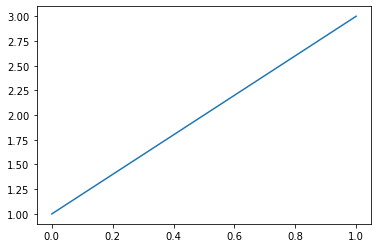Or use an inline code directly: `{{plt.plot([1, 4])}}`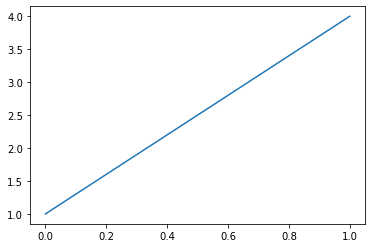### 1.3.4 Auto numbering of headers, figures, tables, etc.

As you can see, all of headers are numbered in this document. This numbering has done by Pheasant automatically. In addition, Pheasant can count the number of figures, tables, etc. and give the identical number to each object.

You can use a special "header" statement for figure, table, etc. to number them like below:

``````#Fig Markdown link for an image can be numbered. {#cat#}
![jpg](img/cat.jpg)``````Figure 1 Markdown link for an image can be numbered.

Supported numbered headers are shown in Table 1:

Table 1 Supported numbered headers

Type Markdown (case-insensitive)
Figure #Figure (title), #Fig (title)
Table #Table (title), #Tab (title)
Equation #Eq (equation), #Eq* (equation)
[other] #[other] (title)

In the above Markdown source, `{#<tag>#}` is an ID tag for hyperlink described below. Off course, you can use any codes to create a figure.

``````#Figure A Matplotlib figure
```python
plt.plot([2, 4])
`````````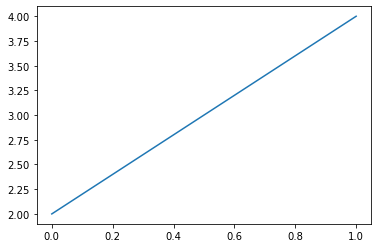Figure 2 A Matplotlib figure

Like figures, tables can be numbered.

``````#Table A Markdown table
a | b
--|--
0 | 1
2 | 3``````

Table 2 A Markdown table

a b
0 1
2 3

Pandas's DataFarme is useful to create a table programmatically.

``````#Table A Pandas's DataFrame
```python
import pandas as pd
pd.DataFrame([[1, 2], [3, 4]], index=list('XY'), columns=list('ab'))
`````````

Table 3 A Pandas's DataFrame

a b
X 1 2
Y 3 4

A plain Markdown source which is not processed by Pheasant has to be separated by a blank line from the following Markdown source which is not a part of the figure or table. If a figure or table has blank lines within it, you have to write the content in a fenced code with tilde (`~~~`).

``````#Fig A figure with a blank line
~~~
![jpg](img/cat.jpg)

![jpg](img/cat.jpg)
~~~``````Figure 3 A figure with a blank line

In addition, Pheasant provides an easy way to number objects regardless of whether they actually have any blank lines or not. Try this:

``#Figure {{plt.plot([1, 5])}} Numbered figure using an inline code.``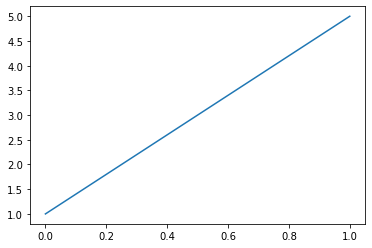Figure 4 Numbered figure using an inline code.

Numbered objects are linked from Markdown source like below:

``For example, go to Fig. {#cat#}``

For example, go to Fig. 1

``#### MkDocs (https://www.mkdocs.org/)``

### 1.3.6 Inspect source (Python only)

`inspect` option to get source.

#### 1.3.6.1 Source code in a code cell

`````````python inspect
def func(x):
return x + 2

func
`````````
``````def func(x):
return x + 2

func?``````

 2020-12-20 08:15:12 (14.4ms) (inspect) python3 (794ms)

``````def func(x):
return x + 2
``````

#### 1.3.6.2 Source code from file

`````````python inspect
from pheasant.renderers.jupyter.jupyter import Cell

Cell
`````````
``````from pheasant.renderers.jupyter.jupyter import Cell

Cell?``````

 2020-12-20 08:15:12 (14.3ms) (inspect) python3 (837ms)

``````@dataclass
class Cell:
code: str
context: Dict[str, str]
template: str
valid: bool = field(default=True, init=False)
cached: bool = field(default=False, compare=False)
output: str = field(default="", compare=False)
extra_module: str = field(default="", compare=False)
``````

#### 1.3.6.3 Inline mode (with custom 'Code' header)

``#Code {{ func # inspect }}``

Code 1

``````def func(x):
return x + 2
``````